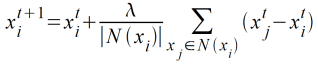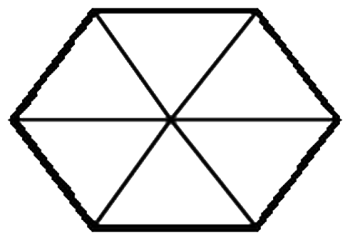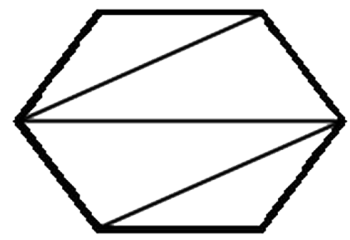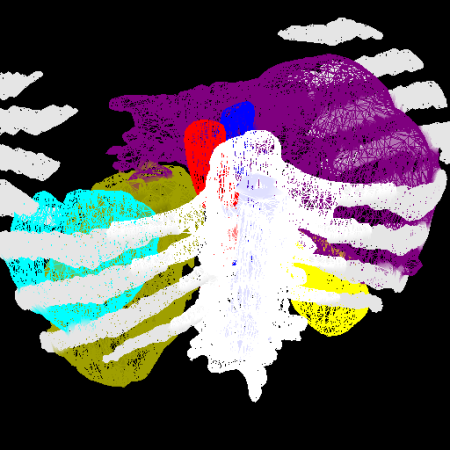# The Legion's Revolting!

The Legion's Revolting!

By Stuart Golodetz

3D meshes can be too large to deal with efficiently. Stuart Golodetz applies some ancient discipline.

In my last article, I explained the first stage of the multiple material marching cubes (M3C) algorithm [ Wu03 ] for generating polygonal meshes from labelled volumes. For my own work in medical image analysis, I make use of this algorithm to generate 3D models from a series of segmented (labelled) CT slices. The meshes produced by this first stage of the process are reasonable, but (as I noted last time) they tend to suffer from 'stair-stepping', due to the inter-slice distance being substantially greater than the intra-slice distance (the distance between the centres of adjacent pixels in a slice). They also contain far too many triangles.

I will discuss solutions to both of these problems in this article. Stair-stepping (and indeed lack of smoothness in general) can be mitigated by a technique called Laplacian smoothing ; excessive triangle counts can be cured by a process known as decimation .

## Stage 2: Laplacian smoothing (overview)

For single-material meshes, Laplacian smoothing is actually a remarkably simple 'averaging' process. The idea is essentially to iteratively move each mesh node a small distance towards its neighbours, using the equation:In other words, the position of x i at iteration t +1 is calculated by adding offset vectors in the directions of each of its adjacent nodes in N ( x i ). The following things are worth noting:

• λ is known as the relaxation factor and can be set by the user: it is usually defined to be somewhere between 0 and 1
• The adjacent nodes don't change between iterations, hence we can write N ( x i ) rather than N ( x i t )
• The equation in [ Wu03 ] misses the division by | N ( x i )|: it turns out to be important

Smoothing multiple-material meshes is a similar process, but with the difference that in this case we only smooth certain nodes in order to preserve the overall topology of the mesh. Nodes can be classified into three types:

• Simple nodes are those that have exactly two material IDs
• Edge nodes are those that have three or more material IDs and are adjacent to exactly two nodes with at least those same material IDs
• Corner nodes are those that have three or more material IDs and are not edge nodes

Having classified the nodes in the mesh, we then smooth the three types differently. Simple nodes are smoothed straightforwardly using the single-material equation given above. Edge nodes (in my implementation) are smoothed similarly, but using a neighbour set consisting of only the two nodes with at least the material IDs of the node being smoothed: note that this differs from the method described in [ Wu03 ], where they restrict edge nodes to motion along the edge path (I tried both, and noticed very little difference in practice). Corner nodes are topologically important and thus not subjected to smoothing at all.

## Stage 2: Laplacian smoothing (implementation)

Implementing Laplacian smoothing in C++ is quite straightforward. The process is initialised with a value for lambda and an iteration count, and the iterate() method is then called the specified number of times on the mesh (see Listing 1, which performs a single iteration of Laplacian smoothing). Each iteration proceeds by classifying each node and then smoothing it according to its classification; all the new positions are copied across en-masse at the end of the iteration because the old positions are required for the smoothing process. The function for actually classifying each node as a simple, edge or corner node is shown in Listing 2.

 ``` template void LaplacianSmoother

 ``` template NodeType classify_node(int i, const std::vector< Node

## Stage 3: Mesh decimation (overview)

In Ancient Roman times, decimation was the barbaric punishment occasionally meted out to rebellious troops, or those who were considered to have shown cowardice in the face of the enemy. A military unit selected for decimation would have its number reduced by a tenth: one in ten men would be selected by lot and clubbed to death by his comrades. The idea was to terrify the remaining troops into obeying orders and fighting courageously: needless to say, however, the process more often reduced morale than increased it. In the case of modern computing, however, decimation is a much more benign procedure. Here, decimation refers to the process of selectively removing triangles and nodes from a mesh until the triangle count has been reduced to the desired level. (The triangle count can often be reduced by as much as 80-90% without greatly affecting the appearance of the mesh!).

There are various different algorithms for this, but the one referred to in [ Wu03 ] works as follows: first of all, a decimation metric is calculated for each simple or edge node that indicates how good a candidate it is for removal from the mesh. For simple nodes, the metric is the perpendicular distance of the node from the average plane of its surrounding loop of adjacent nodes (I described how to calculate the average plane in [ Golodetz08 ]). For an edge node, it is the perpendicular distance from the line joining the two adjacent nodes which have at least the same material IDs [ Wu03 ], but I will only focus on simple nodes for the purposes of this article.

All the nodes are then placed into a 'priority queue' that supports post-insertion updates of element keys (note that std::priority_queue is not such a data structure); the decimation metric for each node is used as its key, so that the first node to be extracted from the queue will be a 'most suitable' candidate for decimation (I say 'a' rather than 'the' because several nodes can be equally good candidates).

Nodes are then iteratively extracted from the queue and the local mesh around them decimated, until some user-defined reduction threshold is reached. In the case of simple nodes, this local decimation process consists of removing the node in question, and all triangles that use it, and retriangulating the loop of adjacent nodes around it using the Schroeder triangulation process introduced in [ Golodetz08 ]. (It is important to note that the adjacent node loop will generally be non-planar, which necessitates a more complicated triangulation scheme than might otherwise be necessary.) The metrics of adjacent nodes are then recalculated, which may cause them to move around in the priority queue.

This process reduces both the triangle and node counts of the mesh. The node count obviously decreases by 1, since a single node has been removed. The triangle count decreases by 2: consider the example of meshing a hexagaon with a central node (6 triangles) compared to one without (4 triangles): see Figure 1.Figure 1

## Stage 3: Mesh decimation (implementation)

The implementation of mesh decimation relies on a peculiar type of priority queue that supports the updating of priorities while elements are still in the queue (see Listing 3). The priority queue itself is represented as a heap (as usual); the only difference is that we also maintain a dictionary to allow elements in the heap to be referenced and their keys modified. In our case, we use each node's global ID as the ID, its decimation metric as the key, and store a mesh decimation functor (to perform the local decimation work) as the data payload.

 ``` template > class PriorityQueue { public: class Element { private: Data m_data; ID m_id; Key m_key; public: Element() {} Element(const ID& id, const Key& key, const Data& data) : m_id(id), m_key(key), m_data(data) {} Data& data() { return m_data; } const ID& id() const { return m_id; } const Key& key() const { return m_key; } friend class PriorityQueue; }; private: // The dictionary maps IDs to their current // position in the heap typedef std::map Dictionary; typedef std::vector Heap; // Datatype Invariant: m_dictionary.size() // == m_heap.size() Dictionary m_dictionary; Heap m_heap; public: void clear() { m_dictionary.clear(); m_heap.swap(Heap()); } bool contains(const ID& id) const { return m_dictionary.find(id) != m_dictionary.end(); } Element& element(const ID& id) { return m_heap[m_dictionary[id]]; } bool empty() const { return m_dictionary.empty(); } void erase(const ID& id) { size_t i = m_dictionary[id]; m_dictionary.erase(id); m_heap[i] = m_heap[m_heap.size()-1]; if(m_heap[i].id() != id) // assuming the // element we were erasing wasn't the last one // in the heap, update the dictionary { m_dictionary[m_heap[i].id()] = i; } m_heap.pop_back(); heapify(i); } void insert(const ID& id, const Key& key, const Data& data) { if(contains(id)) { throw Exception("An element with the specified ID is already in the priority queue"); } size_t i = m_heap.size(); m_heap.resize(i+1); while(i > 0 && Comp()(key, m_heap[parent(i)].key())) { size_t p = parent(i); m_heap[i] = m_heap[p]; m_dictionary[m_heap[i].id()] = i; i = p; } m_heap[i] = Element(id, key, data); m_dictionary[id] = i; } void pop() { erase(m_heap.id()); ensure_invariant(); } Element top() { return m_heap; } void update_key(const ID& id, const Key& key) { size_t i = m_dictionary[id]; update_key_at(i, key); } private: void heapify(size_t i) { bool done = false; while(!done) { size_t L = left(i), R = right(i); size_t largest = i; if(L < m_heap.size() && Comp()(m_heap[L].key(), m_heap[largest].key())) largest = L; if(R < m_heap.size() && Comp()(m_heap[R].key(), m_heap[largest].key())) largest = R; if(largest != i) { std::swap(m_heap[i], m_heap[largest]); m_dictionary[m_heap[i].id()] = i; m_dictionary[m_heap[largest].id()] = largest; i = largest; } else done = true; } } static size_t left(size_t i) { return 2*i + 1; } static size_t parent(size_t i) { // Precondition: i > 0 return (i+1)/2 - 1; } void percolate(size_t i) { while(i > 0 && Comp()(m_heap[i].key(), m_heap[parent(i)].key())) { size_t p = parent(i); std::swap(m_heap[i], m_heap[p]); m_dictionary[m_heap[i].id()] = i; m_dictionary[m_heap[p].id()] = p; i = p; } } static size_t right(size_t i){ return 2*i + 2; } void update_key_at(size_t i, const Key& key) { if(Comp()(key, m_heap[i].key())) { // The key has increased. m_heap[i].m_key = key; percolate(i); } else if(Comp()(m_heap[i].key(), key)) { // The key has decreased. m_heap[i].m_key = key; heapify(i); } } }; ``` Listing 3

The key part of the decimation code, then, is shown in Listing 4. It works as follows: provided there are still undecimated nodes remaining, and we haven't yet reached our reduction target, nodes are repeatedly extracted from the priority queue and the mesh around them decimated. This decimation is handled in practice by marking the node to be decimated as invalid and retriangulating the node loop around it. Some book-keeping is necessary to update the various mesh data structures, after which the new triangles are added to the mesh and the metrics for the surrounding nodes are recalculated. At the end of the process, the triangle list is cleaned by removing any triangles which mention invalid nodes. The node array is then cleaned by removing any nodes which are invalid: this involves renumbering all the remaining nodes, so a map is created from old to new indices, and this is used to update the triangle list accordingly.

 ``` template typename MeshDecimator

Figure 2 shows the end result.Figure 2

## Summary

In this article, we have seen how to smooth and decimate the intermediate meshes produced by the first stage of the multiple material marching cubes (M3C) algorithm [ Wu03 ]. This allows us to generate smooth, reasonably-sized meshes that are suitable for real-time visualization. In the medical imaging domain, these meshes can be used to allow doctors to view a patient's state from any angle (and indeed to 'fly around' the patient's model), greatly aiding their understanding of the 3D situation involved.

## References

[ Golodetz08] Golodetz, SM, Seeing Things Differently, Overload 86, August 2008.

[ Wu03] Wu, Z, and Sullivan Jr., JM, Multiple material marching cubes algorithm, International Journal for Numerical Methods in Engineering, 2003.